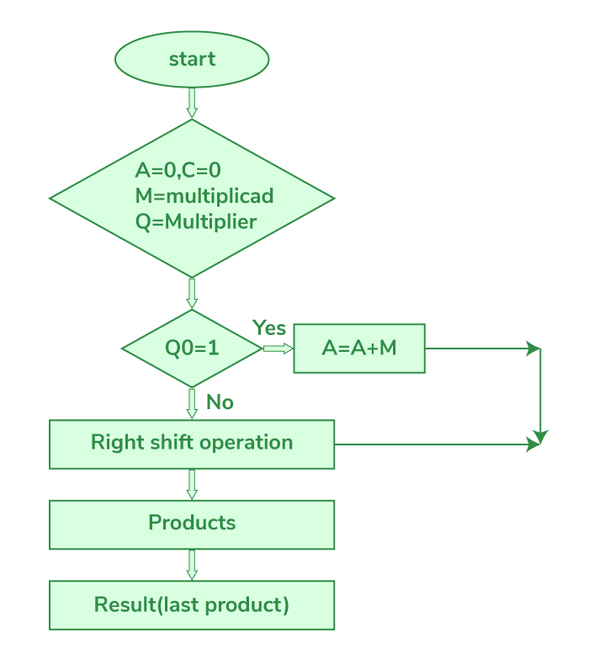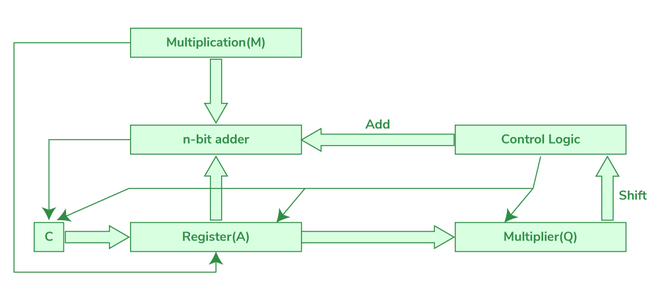Open in App
Not now

# Sequential Binary Multiplier

• Difficulty Level : Basic
• Last Updated : 04 Jul, 2022

In this article, we are going to learn how a sequential binary multiplier works with examples. So for that, we also need to learn a few concepts related to the sequential circuit, binary multipliers, etc. Finally solving the examples using a sequential binary multiplier method.

### Sequential circuit:

A sequential circuit is a combinational circuit with memory. The output of the sequential circuit depends on present inputs and present state [past outputs]. The information stored in the sequential circuit represents the present state. The present state and present input will define the output of the next state.

### Binary multiplier:

A binary multiplier is used to multiply two binary numbers. It is a basic electronic circuit in digital electronics, such as a computer. The binary multiplier is also called an add-shift adder.

A digital multiplier can be implemented using a variety of computer arithmetic techniques. The majority of techniques involve computing a set of partial products, which are then summed using binary adders.

Adder: An adder, also known as summer, is a digital circuit that performs number addition. Adders are used in the arithmetic logic units of many computers and other types of processors (ALUs).

### Types of multipliers:

1. Bit Multiplier 2×2: This multiplier can multiply two numbers with bit size = 2, which means that both the multiplier and the multiplicand can be of 2 bits.
2. Bit Multiplier 3×3: This multiplier has a maximum bit size of 3 bits and can multiply two numbers. The product’s bit size will be 6.
3. Bit Multiplier 4×4: This multiplier can multiply a 4-bit binary number and produce an 8-bit product because the bit size of the product equals the sum of the bit sizes of the multiplier and multiplicand.

Before going to sequential binary multiplication first let’s see how normal binary multiplication works:

### Binary multiplication:

Consider two binary numbers num1 and num2

```num1=12 ⇢ which equivalent to binary value as 1100 ⇢ multiplier
num2=13 ⇢ which equivalent to binary value as 1101 ⇢ multiplicand```

Example 1:

```                  1 1 0 0
x 1 1 0 1
----------------------
1 1 0 0
0 0 0 0
1 1 0 0
1 1 0 0
--------------------
1 0 0 1 1 1 0 0```

The above multiplication is performed by a sequential binary multiplier as below:

Operation:

Consider two binary numbers num1 and num2

Step 1:

```num1=12 ⇢ which equivalent to a binary value as 1100
num2=13 ⇢ which equivalent to binary value as 1101 ```

Assume multiplier as M and multiplicand as Q

Step 2:

```Here we also need the other two parameters accumulator and carry and
initially the values of both accumulator and carry will be zero.
Let,
Accumulator a = 0000
Carry c = 0  ```

Step 3:

```Q = 1  1  0  1
q3 q2 q1 q0 ```

if q0=0 then perform an only right shift operation

if q0=1 then perform add (A+M) and  right shift operation

Here we have to perform up to 4 steps because the number of bits in the multiplier is 4

Step 4:

```Result = Combination of accumulator value(A) and Q =>10011100
the equivalent value is 156 obtained from the formula
.........+23+22+21+20
1   0   0   1    1   1    0   0
27+0+0+24+23+22+0+0 =156```

### Flow chart:

The flow chart explains the whole operation of the sequential binary multiplier in a simple manner. First, assign 0 to the accumulator and carry values. then check the LSB of Q i.e Q0, if q0 is 0 then perform only the right shift operation and if q0 is 1 then perform the addition of the accumulator and multiplicand, store the result in the accumulator then perform the right shift operation. We have to continue this process based on the number of bits in the multiplier.Flow chart of sequential binary multiplier

### Hardware circuit diagram:

The below diagram describes the hardware circuit of the sequential binary multiplier.Hardware circuit diagram

Example 2: Here we have to perform up to 4 steps because the number of bits in the multiplier is 4.

Result:

```Combination of accumulator value(A) and Q =>01010100
the equivalent value is 84 obtained from the formula
.........+23+22+21+20
0   1    0   1    0   1   0  0
0+64+0+16+0+8+0+0 ⇢ 84```

My Personal Notes arrow_drop_up
Related Articles